Courses

# Inequalities CA Foundation Notes | EduRev

## Quantitative Aptitude for CA CPT

Created by: Wizius Careers

## CA Foundation : Inequalities CA Foundation Notes | EduRev

The document Inequalities CA Foundation Notes | EduRev is a part of the CA Foundation Course Quantitative Aptitude for CA CPT.
All you need of CA Foundation at this link: CA Foundation

INEQUALITIES

LEARNING OBJECTIVES
One of the widely used decision making problems, nowadays, is to decide on the optimal mix of scarce resources in meeting the desired goal. In simplest form, it uses several linear inequations in two variables derived from the description of the problem.
The objective in this section is to make a foundation of the working methodology for the above by way of introduction of the idea of :

• development of inequations from the descriptive problem;
• graphing of linear inequations; and
• determination of common region satisfying the inequations.

3.1  INEQUALITIES

Inequalities are statements where two quantities are unequal but a relationship exists between them. These type of inequalities occur in business whenever there is a limit on supply, demand, sales etc. For example, if a producer requires a certain type of raw material for his factory and there is an upper limit in the availability of that raw material, then any decision which he takes about production should involve this constraint also. We will see in this chapter more about such situations.

3.2 LINEAR INEQUALITIES IN ONE VARIABLE AND THE SOLUTION SPACE
Any linear function that involves an inequality sign is a linear inequality. It may be of one variable, or, of more than one variable. Simple example of linear inequalities are those of one variable only; viz., x > 0, x < 0 etc.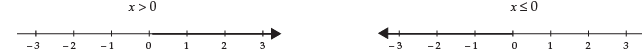The values of the variables that satisfy an inequality are called the solution space, and is abbreviated as S.S. The solution spaces for (i) x > 0,  (ii) x ≤ 0 are shaded in the above diagrams, by using deep lines.
Linear inequalities in two variables: Now we turn to linear inequalities in two variables x and y and shade a few S.S.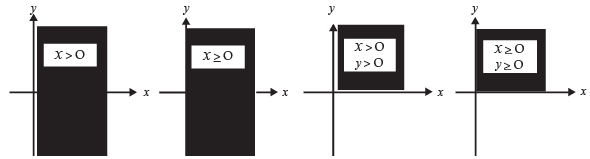Let us now consider a linear inequality in two variables given by 3x + y < 6

The inequality mentioned above is true for certain pairs of numbers (x, y) that satisfy 3x + y < 6. By trial, we may arbitrarily find such a pair to be (1,1) because 3 × 1 + 1 = 4, and 4 < 6.
Linear inequalities in two variables may be solved easily by extending our knowledge of straight lines.
For this purpose, we replace the inequality by an equality and seek the pairs of number that satisfy 3x + y = 6. We may write 3x + y = 6 as y = 6 – 3x, and draw the graph of this linear function.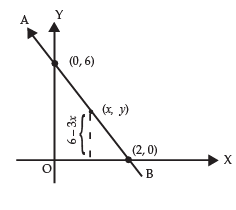Let x = 0 so that y = 6. Let y = 0, so that x = 2.
Any pair of numbers (x, y) that satisfies the equation y = 6 – 3x falls on the line AB.
Note: The pair of inequalities x ≥ 0, y ≥ 0 play an important role in linear programming problems.
Therefore, if y is to be less than 6 – 3x for the same value of x, it must assume a value that is less than the ordinate of length 6 – 3x.
All such points (x, y) for which the ordinate is less than 6 – 3x lie below the line AB.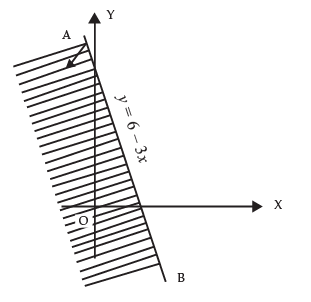The region where these points fall is indicated by an arrow and is shaded too in the adjoining diagram. Now we consider two inequalities 3x + y ≤ 6 and  x – y ≤ –  2 being satisfied simultaneously by x and y. The pairs of numbers (x, y) that satisfy both the inequalities may be found by drawing the graphs of the two lines y = 6 – 3x and y = 2 + x, and determining the region where both the inequalities hold. It is convenient to express each equality with y on the left-side and the remaining terms in the right side. The first inequality 3x + y ≤ 6 is equivalent to y ≤ 6 – 3x and it requires the value of y for each x to be less than or equal to that of and on 6 – 3x. The inequality is therefore satisfied by all points lying below the line y = 6 – 3x. The region where these points fall has been shaded in the adjoining diagram.
We consider the second inequality x – y ≤  –2, and note that this is equivalent to y ≥ 2 + x. It requires the value of  y  for each x to be larger than or equal to that of  2 + x. The inequality is, therefore, satisfied by all points lying on and above the line y = 2 + x.
The region of interest is indicated by an arrow on the line y = 2 + x in the diagram below.
For x = 0, y = 2 + 0 = 2;
For y = 0, 0 = 2 + x   i.e,   x = –2.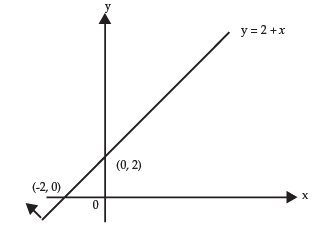By superimposing the above two graphs we determine the common region ACD in which the pairs (x, y) satisfy both inequalities.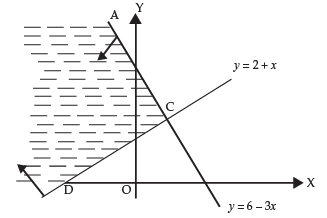We now consider the problem of drawing graphs of the following inequalities x ≥ 0,  y ≥ 0,  x ≤ 6,  y ≤ 7,   x + y ≤ 12 and shading the common region.

Note:  The inequalities 3x + y ≤ 6 and x – y ≤ 2 differ from the preceding ones in that these also include equality signs. It means that the points lying on the corresponding lines are also included in the region.
 The procedure may be extended to any number of inequalities.

We note that the given inequalities may be grouped as follows :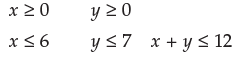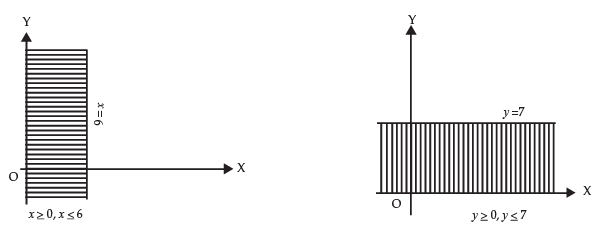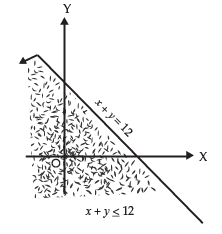By superimposing the above three graphs, we determine the common region in the  xy plane where all the five inequalities are simultaneously satisfied.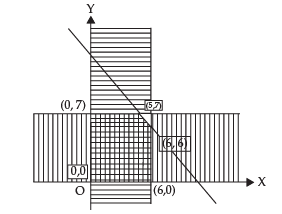Example: A company produces two products A and B, each of which requires processing in two machines. The first machine can be used at most for 60 hours, the second machine can be used at most for 40 hours. The product A requires 2 hours on machine one and one hour on machine two. The product B requires one hour on machine one and two hours on machine two. Express above situation using linear inequalities.

Solution: Let the company produce, x number of product A and y number of product B. As each of product A requires 2 hours in machine one and one hour in machine two, x number of product A requires 2x hours in machine one and x hours in machine two. Similarly, y number of product B requires y hours in machine one and 2y hours in machine two. But machine one can be used for 60 hours and machine two for 40 hours. Hence  2x + y  cannot exceed 60 and x +2y cannot exceed 40. In other words,

Solution: Let the company produce, x number of product A and y number of product B. As each of product A requires 2 hours in machine one and one hour in machine two, x number of product A requires 2x hours in machine one and x hours in machine two. Similarly, y number of product B requires y hours in machine one and 2y hours in machine two. But machine one can be used for 60 hours and machine two for 40 hours. Hence  2x + y  cannot exceed 60 and x +2y cannot exceed 40. In other words,

2x + y ≤ 60 and   x + 2y ≤ 40.

Thus, the conditions can be expressed using linear inequalities.

Example: A fertilizer company produces two types of fertilizers called grade I and grade II.
Each of these types is processed through two critical chemical plant units. Plant A has maximum of 120 hours available in a week and plant B has maximum of 180 hours available in a week.
Manufacturing one bag of grade I fertilizer requires 6 hours in plant A and 4 hours in plant B.
Manufacturing one bag of grade II fertilizer requires 3 hours in plant A and 10 hours in plant B.
Express this using linear inequalities.

Solution: Let us denote by x1, the number of bags of fertilizers of grade I and by x2, the number of bags of fertilizers of grade II produced in a week. We are given that grade I fertilizer requires 6 hours in plant A and grade II fertilizer requires 3 hours in plant A and plant A has maximum of 120 hours available in a week. Thus  6x+ 3x2 ≤ 120.
Similarly grade I fertilizer requires 4 hours in plant B and grade II fertilizer requires 10 hours in Plant B and Plant B has maximum of 180 hours available in a week. Hence, we get the inequality 4x1 + 10x2 ≤ 180.

Example: Graph the inequalities 5x1 + 4x2 ≥ 9,    x1 + x2 ≥ 3,  x1 ≥ 0   and   x2 ≥ 0 and mark the common region.

Solution: We draw the straight lines 5x1 + 4x2 = 9 and x1 + x2 = 3.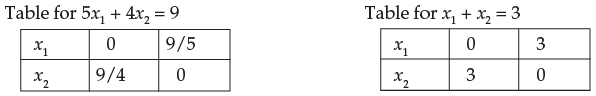Now, if we take the point (4, 4), we find
5x1 + 4x2 ≥ 9
i.e., 5.4 + 4.4 ≥ 9
or, 36 ≥ 9 (True)
x1 + x2 ≥ 3
i.e., 4 + 4 ≥ 3
8 ≥ 3 (True)

Hence (4, 4) is in the region which satisfies the inequalities.
We mark the region being satisfied by the inequalities and note that the cross-hatched region is satisfied by all the inequalities.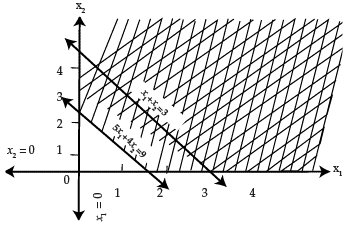Example: Draw the graph of the solution set of the following inequality and equality:
x + 2y = 4.
x – y ≤ 3.
Mark the common region.

Solution: We draw the graph of both x + 2y = 4 and x – y ≤ 3 in the same plane.
The solution set of system is that portion of the graph of x + 2y = 4 that lies within the half-plane representing the inequality x – y ≤ 3.
Solution: We draw the graph of both x + 2y = 4 and x – y ≤ 3 in the same plane.
The solution set of system is that portion of the graph of x + 2y = 4 that lies within the half-plane representing the inequality x – y ≤ 3.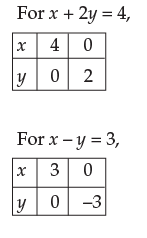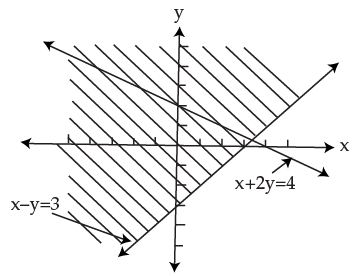Example: Draw the graphs of the following inequalities:
x + y ≤ 4,
x – y ≤ 4,
x ≥ –2.
and mark the common region.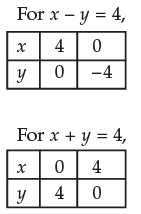The common region is the one represented by overlapping of the shadings.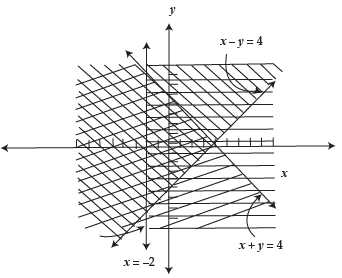Example: Draw the graphs of the following linear inequalities: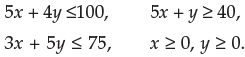and mark the common region.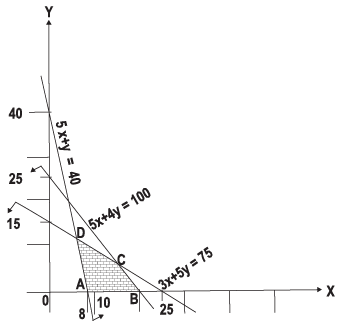Solution: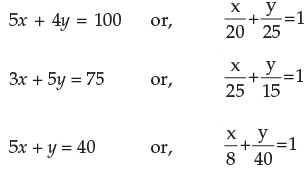Plotting the straight lines on the graph paper we have the above diagram:
The common region of the given inequalities is shown by the shaded portion ABCD.

Example: Draw the graphs of the following linear inequalities:
5x + 8y ≤ 2000, x ≤ 175, x ≥ 0.
7x + 4y ≤ 1400, y ≤ 225, y ≥ 0.
and mark the common region:

Solution: Let us plot the line AB (5x +8y = 2,000) by joining the points A(400, 0) and B(0, 250).
Similarly, we plot the line CD (7x + 4y = 1400) by joining the points C(200, 0) and D(0, 350).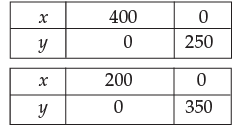Also, we draw the lines EF(x = 175) and GH (y = 225).
The required graph is shown alongside in which the common region is shaded.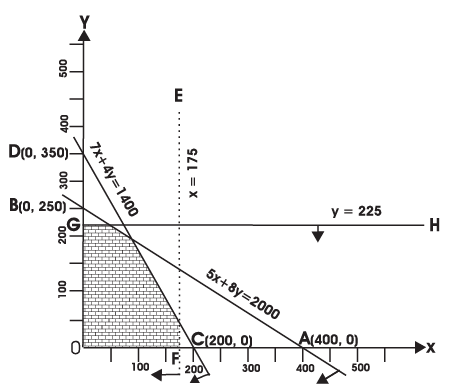Example:   Draw the graphs of the following linear inequalities: x + y ≥ 1, 7x + 9y ≤ 63,
y ≤  5, x ≤ 6,x ≥ 0,  y ≥ 0. and mark the common region.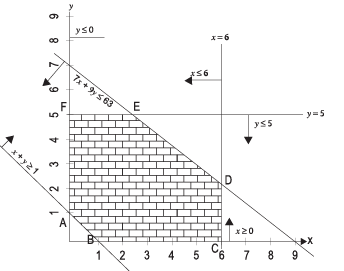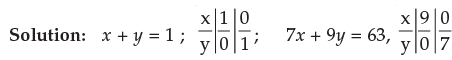We plot the line AB (x + y = 1), CD (y = 5), EF (x = 6), DE (7x + 9y = 63).
Given inequalities are shown by arrows.
Common region ABCDEF is the shaded region.

Example: Two machines (I and II) produce two grades of plywood, grade A and grade B. In one hour of operation machine I produces two units of grade A and one unit of grade B, while machine II, in one hour of operation produces three units of grade A and four units of grade B.
The machines are required to meet a production schedule of at least fourteen units of grad A and twelve units of grade B. Express this using linear inequalities and draw the graph.

Solution: Let the number of hours required on machine I be x and that on machine II be y.
Since in one hour, machine I can produce 2 units of grade A and one unit of grade B, in x hours it will produce 2x and x units of grade A and B respectively. Similarly, machine II, in one hour, can produce 3 units of grade A and 4 units of grade B. Hence, in y hours, it will produce 3y and 4y units Grade A & B respectively.
The given data can be expressed in the form of linear inequalities as follows:
2x + 3y ≥ 14 (Requirement of grade A)
x + 4y ≥ 12 (Requirement of grade B)
Moreover x and y cannot be negative, thus x ≥ 0 and y ≥ 0
Let us now draw the graphs of above inequalities. Since both x and y are positive, it is enough to draw the graph only on the positive side.
The inequalities are drawn in the following graph: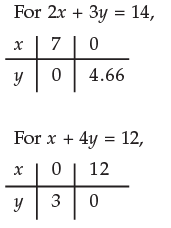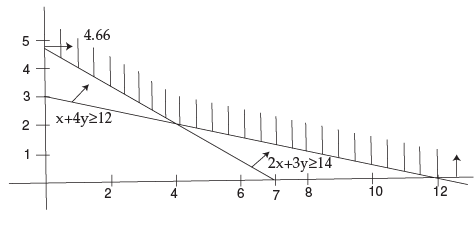'

In the above graph we find that the shaded portion is moving towards infinity on the positive side. Thus the result of these inequalities is unbounded.

66 docs|68 tests

,

,

,

,

,

,

,

,

,

,

,

,

,

,

,

,

,

,

,

,

,

;